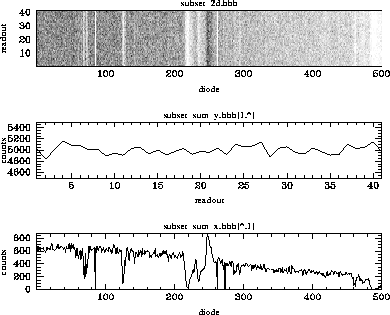[Top] [Prev] [Next] [Bottom]

# 35.7 RAPID Mode (and a Little About Spatial Scans)

RAPID mode was used relatively rarely, but it had some unique capabilities. There are also some unique problems that arise in treating the resultant data.

## 35.7.1 The Exposure Logsheet

An abridged version of the Phase II Proposal for 5745 is shown in Figure 35.18. Here are some notes to go with individual lines of the Exposure Logsheet:

#### Figure 35.18: Exposure Logsheet for Program 5745

```

```
------------------------------------------------------------------------------------------------------------------------------------

## 35.7.2 Examining the RAPID Data

First let's look to see how many individual spectra we have:

```cl> imhead ../data/z2i3010at.d0h,../data/z2i3010at.c1h l-
../data/z2i3010at.d0h[1/9024][real]: Z2I3010AT[1/9024]
../data/z2i3010at.c1h[1/9021][real]: Z2I3010AT[1/9021]

```
The imheader task shows that we have many more spectra than expected. Further investigation turned up the fact that ten seconds was allocated for the slew from dwell point to dwell point. So we ended up having an extra ten seconds for each slew. Not all of these extra spectra are useful since the telescope was actually moving, but we ended up getting about 35 seconds per dwell point instead of the anticipated 30 seconds. The imheader output also shows that there are three less spectra in the calibrated output than in the raw data. Keep in mind that since there is no substepping or FP-SPLITs possible when using RAPID mode, that a single raw spectrum maps to a single calibrated spectrum. (Contrast this with a standard ACCUM with the default STEP-PATT: the raw data would contain six individual spectra-four substeps and two background spectra-which would be merged into a single calibrated spectrum.) In the case of RAPID mode, the first two readouts were generated during a hysteresis sequence that preceded every observation, and the last readout was produced by a final pass deflection made at the end of every observation. These readouts contain no useful science data and are not included in the calibrated data.

Manipulating a very large image can be difficult. For demonstration purposes, let's examine an arbitrary subsection of the original raw image in Figure 35.20. The gcopy task was used to extract a subset of groups from the original multi-group image. gstat computed the statistics for the new image.

```cl> gftoxdim subset.hhh subset_d2.hhh

```
The new image is now two-dimensional: the first dimension runs from diode 1 to 500 while the second dimension marks time.

```cl> imhead subset_d2.hhh l-
subset_2d.hhh[500,41][real]: SUBSET_2D[1/1]
cl> display subset_d2.hhh 1 z1=0 z2=40 zr- zs-

```

#### Figure 35.20: Manipulating a Large Image

```
cl> gcopy ../data/z2i3010at.d0h subset.hhh groups=4604-4644

```
```../data/z2i3010at.d0h -> subset.hhh

```
```cl> gstat subset.hhh groups=*

```
```# Image Statistics for subset.hhh

```
```# GROUP       NPIX      MEAN     MIDPT    STDDEV       MIN       MAX

```
```     1         500    10.048   9.00849    5.7949        0.       28.

```
```     2         500      9.68   9.87606   5.31584        0.       24.

```
```     3         500       10.   9.12097   5.48161        0.       27.

```
```     4         500    10.334   9.88548   5.68217        0.       27.

```
```     5         500    10.174   9.94917   5.75412        0.       28.

```
```     6         500    10.166   9.00516   5.83254        0.       28.

```
```     7         500    10.014   9.06106   5.56919        0.       25.

```
```     8         500     10.04    9.8603   5.64465        0.       29.

```
```     9         500     9.792   9.00807   5.29384        0.       28.

```
```    10         500     9.886   9.92473   5.40587        0.       26.

```
```    11         500     9.818   8.99274   5.39881        0.       28.

```
```    12         500    10.072   9.82091   5.79603        0.       27.

```
```    13         500    10.092   9.89125   5.59913        0.       25.

```
```    14         500     9.856   9.10092   5.50562        0.       29.

```
```    15         500    10.004   9.05718   5.64195        0.       29.

```
```    16         500     9.822   9.01055   5.71381        0.       35.

```
```    17         500     9.952   9.88586   5.57313        0.       24.

```
```    18         500    10.054   9.94277   5.68822        0.       30.

```
```    19         500     9.856   9.01978   5.35805        0.       25.

```
```    20         500     9.958   8.97168   5.75312        0.       28.

```
```    21         500     9.916   8.98258   5.69824        0.       26.

```
```    22         500     10.07   9.86902   5.49741        0.       23.

```
```    23         500     9.834   9.90083   5.55231        0.       25.

```
```    24         500    10.206   9.83871   5.75446        0.       32.

```
```    25         500    10.122   9.00645   5.72859        0.       28.

```
```    26         500    10.148    10.128   5.65137        0.       31.

```
```    27         500    10.286   10.0233    5.7052        0.       28.

```
```28 500      9.76   9.03122   5.41859        0.       25.

```
```29         500    10.068   9.04799   5.69493        0.       25.

```
```30         500     10.12   10.0108   5.52473        0.       28.

```
```31         500     9.908   8.98854   5.53071        0.       26.

```
```32         500     9.856   9.09568   5.40905        0.       29.

```
```33         500    10.076   9.90533   5.61045        0.       25.

```
```34         500     9.942   9.84996   5.42379        0.       27.

```
```35         500     9.822   9.88937   5.39598        0.       25.

```
```36         500     9.832   9.87903   5.58553        0.       25.

```
```37         500    10.206    9.8606   5.54952        0.       27.

```
```38         500     10.05    9.8626   5.61128        0.       26.

```
```39         500    10.102   9.97581   5.49509        0.       25.

```
```40         500    10.304   9.91161   5.80646        0.       27.

```
```41         500     9.926   8.99917   5.49809        0.       32.

```

```cl> blkavg subset_2d.hhh subset_sum_y.hhh b1=1 b2=41 option=sum
cl> blkavg subset_2d.hhh subset_sum_x.hhh b1=500 b2=1 option=sum
cl> imhead subset_sum_?.hhh l-
subset_sum_x.hhh[500,1][real]: SUBSET_SUM_X[1/1]
subset_sum_y.hhh[1,41][real]: SUBSET_SUM_Y[1/1]

```
Figure 35.21 shows plots of three images. At the top is a greyscale plot of the extracted two-dimensional image, in the middle is a plot of the sum of the 500 diodes as a function of time, and at the bottom is a summed plot of the spectra over time.

#### Figure 35.21: RAPID Data Displays[Top] [Prev] [Next] [Bottom]

stevens@stsci.edu
Copyright © 1997, Association of Universities for Research in Astronomy. All rights reserved. Last updated: 01/14/98 15:45:39